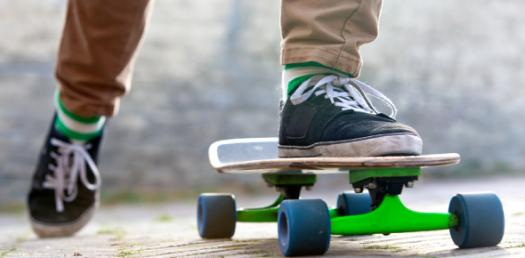# Physics Quiz: Force And Motion Questions!

12 Questions | Total Attempts: 3412Settings.

• 1.
Which best describes the effect of force on the motion of an object?
• A.

Force causes the object to change shape or mass.

• B.

Force causes the object to change speed or direction.

• C.

Force causes the object to experience gravity.

• D.

Force causes the object to experience balance.

• 2.
Sequence the graphs in order from least to greatest speed.   (Slowest to fastest)
• A.

C, D, B, A

• B.

A, B, D, C

• C.

A, B, C, D

• D.

C, A, B, D

• 3.
Pushes and pulls are ___
• A.

Weights

• B.

Forces

• C.

Balanced

• D.

Matter

• 4.
What causes an object's motion to change speed?
• A.

Size and shape

• B.

Volume

• C.

Forces

• D.

Mass

• 5.
• A.

Thermometer

• B.

Triple beam balance

• C.

• D.

Spring scale

• 6.
Which example represents balanced forces?
• A.

Ball rolling down hill

• B.

Apple falling from tree

• C.

When there is no changes in motion

• D.

One football player is stronger than the other

• 7.
Which statement(s) are true
• A.

Box B is pushed a greater distance because the applied force is greater.

• B.

More work is done on box B because the force and distance is greater.

• C.

The greater the amount of force applied to the box, the further the distance it is pushed.

• D.

All are true

• 8.
When equal forces act on an object in opposite directions___
• A.

The object will change its motion because the forces are unbalanced

• B.

The object will stay the same because the forces are balanced

• 9.
What is the advantage of using a pulley?
• A.

It reduces the amount of force needed

• B.

The string has to be pulled a greater distance

• C.

When a pulley is used, no work is done

• 10.
• A.

A

• B.

B

• C.

C

• D.

D

• 11.
The inclined plane (ramp) decreases the input force by increasing the ___
• A.

Energy

• B.

Direction

• C.

Distance

• D.

Work

• 12.
Which portion of the graph represents the turtle stopping to rest?
• A.

Q

• B.

X

• C.

Z

• D.

Y

Related TopicsBack to top